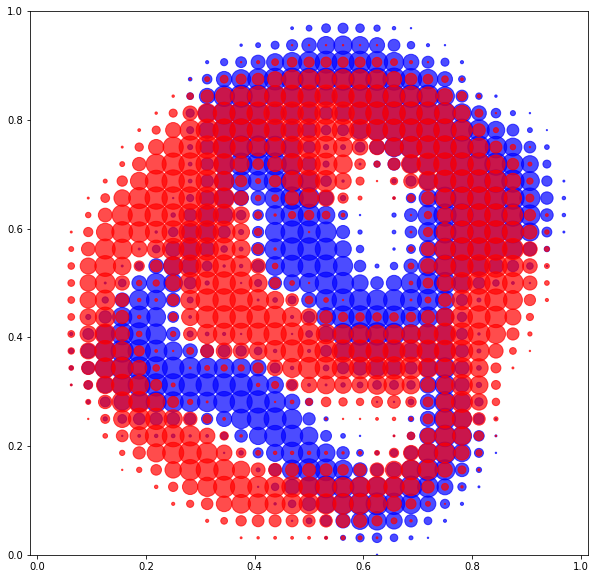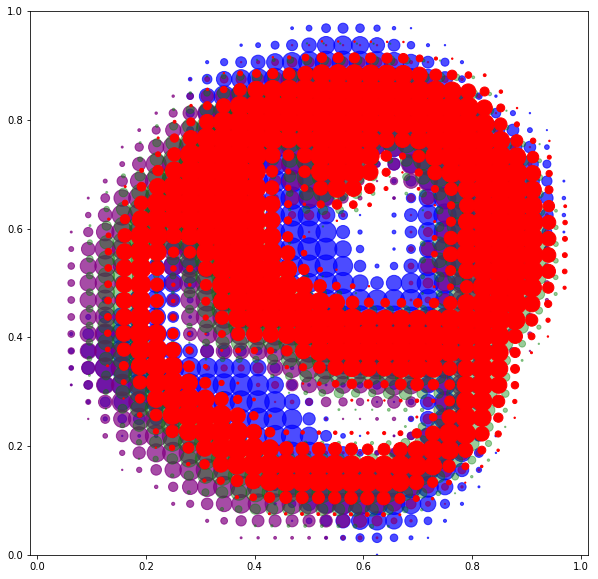# 1) Working with unlabeled data¶

In :
%matplotlib inline
import matplotlib.pyplot as plt

# Mandatory imports...
import numpy as np
import torch
from torch import tensor
from torch.nn import Parameter

# Custom modules:
from model import Model  # Optimization blackbox
from display import plot # Simple plotting routine for measures
from torch_complex import rot # Rotations in 2D


Outline. In yesterday's session, we've seen how to perform shape analysis on labeled point clouds. In practice, though, most datasets are unlabeled and we do not know a good mapping (or registration) between the source and the target.

In this notebook, we present a simple fidelity, or data attachment term that allows us to work with unlabeled shapes. Needless to say, this approach can be used in conjunction with a set of reference landmarks position given by a features detector: one just needs to sum the unlabeled fidelity and the standard $L^2$ distance between the landmarks.

## A) Encoding shapes as measures¶

Intensity vs Density. In computational anatomy, we're mostly working with two types of data: images (intensity volumes) and segmentation maps (density volumes, curves or surface meshes). Medical images pose specific challenges (signal drift in MRIs, huge memory footprint...) and we do not intend to adress them in this introductory session.

In today's workshop, we will thus focus on the (mathematically) cleaner setting of segmentation maps and work with grayscale bitmaps $A$ and $B$ that represent densities of a specific tissue in the 2D plane. Mathematically, we can think of $A$ and $B$ as measures $\alpha$ and $\beta$, and write them as sums of atomic Dirac masses:

\begin{align} \alpha~&=~\sum_{i=1}^N \alpha_i\,\delta_{x_i}, & \beta~&=~\sum_{j=1}^M \beta_j\,\delta_{y_j}. \end{align}

Here, $(x_i)$ and $(y_j)$ are encoded through real valued N-by-2 and M-by-2 tensors while $(\alpha_i)$ and $(\beta_j)$ are given as N-by-1 and M-by-1 positive vectors. For the sake of simplicity, we will normalize both segmentation maps:

\begin{align} \sum_{i=1}^N \alpha_i ~~=~~1~~=~~\sum_{j=1}^M \beta_j. \end{align}

In :
from sampling import load_measure

# Images from the Spectral Log-Demons paper (2013, Lombaert et Al.)
print("Number of atoms : N={} and M={}.".format(len(α), len(β)))

Number of atoms : N=630 and M=596.


Restricting ourselves to operations that are well-defined on measures allows us to guarantee sampling and parametrization-invariance in our algorithms.

Since we're working on your laptop's CPU, we use tiny images... let's display them in the unit square:

In :
plt.figure(figsize=(10,10))
plot(β, "blue", alpha=.7)
plot(α, "red", alpha=.7)
plt.axis("equal")
plt.axis([0,1,0,1])
plt.show()Global divergences. To compare probability distributions with varying supports, the simplest method is to pick a conditionally positive, universal kernel $k:\mathbb{R}^2\rightarrow\mathbb{R}$ and to use

\begin{align} \tfrac{1}{2}\|\alpha-\beta\|_k^2 ~=~ \tfrac{1}{2}\langle \alpha - \beta, k\star(\alpha-\beta)\rangle. \end{align}

as a squared norm between the sampled measures. Here, $\star$ is the standard, linear convolution product so that for instance

\begin{align} \langle\alpha, k\star\beta\rangle ~=~ \sum_{i=1}^N\sum_{j=1}^M \alpha_i\beta_j\,k(x_i-y_j) ~=~ \alpha_i^\top\,K_{x_iy_j}\,\beta_j, \end{align} where $K_{x_iy_j}$ is the kernel matrix associated to $(x_i)$ and $(y_j)$.

Unfortunately, standard machine-learning kernels are unfit for the registration of sampled shapes. The Gaussian RBF kernel $\exp(-\|x-y\|^2/2\sigma^2)$ for instance, has nothing but weaknesses in this setting: its smoothness means that it is blind to high-frequency oscillations; its compact support, that the norm's gradient with respect to the $x_i$'s vanishes away from the support of $\beta$.

In practice (as advocated in Global divergences between measures (2018), Feydy, Trouvé), the scale-invariant Energy Distance kernel from statistics

\begin{align} k(x-y)~~=~~ -\|x-y\| \end{align}

provides a much more robust baseline: it is pointy, has global support and provides well-behaved gradients.

In :
def scal( f, g ) :
"Scalar product between two vectors."

def sqdistances(x_i, y_j) :
"Matrix of squared distances, C_ij = |x_i-y_j|^2."
return ( (x_i.unsqueeze(1) - y_j.unsqueeze(0)) ** 2).sum(2)

def distances(x_i, y_j) :
"Matrix of distances, C_ij = |x_i-y_j|."
return (x_i.unsqueeze(1) - y_j.unsqueeze(0)).norm(p=2,dim=2)

def fidelity(α, β) :
"Energy Distance between two sampled probability measures."
α_i, x_i = α
β_j, y_j = β
K_xx = -distances(x_i, x_i)
K_xy = -distances(x_i, y_j)
K_yy = -distances(y_j, y_j)
cost = .5*scal( α_i, K_xx @ α_i ) \
-    scal( α_i, K_xy @ β_j ) \
+ .5*scal( β_j, K_yy @ β_j )
return cost


## B) Rigid registration¶

Equipped with a parametrization-invariant fidelity, we can now register a measure onto another. Using, for instance, the similarity group:

In :
class RigidRegistration(Model) :
"Find the optimal translation, scaling and rotation."
def __init__(self, α) :
"Defines the parameters of a rigid deformation of α."
super(Model, self).__init__()
self.α, self.x = α.detach(), α.detach()
self.λ = Parameter(tensor(  0.     )) # log-Scale
self.θ = Parameter(tensor(  0.     )) # Angle
self.τ = Parameter(tensor( [0.,0.] )) # Position

def __call__(self, t=1.) :
# At the moment, PyTorch does not support complex numbers...
x_t = (t*self.λ).exp()*rot(self.x, t*self.θ) + t*self.τ
return self.α, x_t

def cost(self, target) :
"Returns a cost to optimize."
return fidelity( self(), target)


To understand the trajectory from $\alpha$ to $\beta$, we display the model at intermediate timesteps:

In :
def train_and_display(Model, source, target) :
# Model: orbit of the source
model = Model( source )
model.fit( target ) # Fit to the target, scikit-learn like

plt.figure(figsize=(10,10))
plot(target, "blue", alpha=.7)
plot(source, "purple", alpha=.7)
for t in [.5] : plot(model(t), "green", alpha=.4)
plot(model(), "red")
plt.axis("equal")
plt.axis([0,1,0,1])

plt.figure(figsize=(10,3))
plt.subplot(1,3,1)
plot(target, "blue",   alpha=.4, scale=.1)
plot(source, "purple", alpha=.4, scale=.1)
plt.axis("equal")
plt.axis([0,1,0,1])

plt.subplot(1,3,2)
plot(target,    "blue",  alpha=.4, scale=.1)
plot(model(.5), "green", alpha=.4, scale=.1)
plt.axis("equal")
plt.axis([0,1,0,1])

plt.subplot(1,3,3)
plot(target, "blue", alpha=.4, scale=.1)
plot(model(), "red", alpha=.4, scale=.1)
plt.axis("equal")
plt.axis([0,1,0,1])

plt.show()

return model


Let's see how it goes!

In :
rigid = train_and_display(RigidRegistration, α, β)

It  1:0.004. It  2:0.007. It  3:0.002. It  4:0.002. It  5:0.002.
It  6:0.001. It  7:0.001. It  8:0.001. It  9:0.001. It 10:0.001.

b'CONVERGENCE: REL_REDUCTION_OF_F_<=_FACTR*EPSMCH'